# Validation functions

Tags

From the last lesson, here's the code to validate price:

• // Price.
• \$priceErrorMessage = '';
• if (!isset(\$_GET['price'])) {
•     \$priceErrorMessage = 'Sorry, you must give a price.<br>';
• }
• // Numeric check.
• if (\$priceErrorMessage == '') {
•     // Get the price.
•     \$price = \$_GET['price'];
•     // Check that price is numeric.
•     if (!is_numeric(\$price)) {
•         \$priceErrorMessage = 'Sorry, price must be a number.<br>';
•     }
• }
• // Range check.
• if (\$priceErrorMessage == '') {
•     if (\$price <= 0) {
•         \$priceErrorMessage = 'Sorry, price must be more than zero.<br>';
•     }
•     if (\$price >= 1000000) {
•         \$priceErrorMessage = 'Sorry, price is too high to be real.<br>';
•     }
• }

How many pages in a business web app might want to validate prices?Ethan

Well, maybe a lot. Dozens.

Right. It would be a pain to write the code for every page.Ray

Yeah, but we could just copy-and-paste the code to every page.

True. Could that cause any problems?If the code changed, we'd have to change it on every page.

Aye! If we wanted to change the error messages, for example, we might have to touch dozens of pages. That's not only a lot of work, but we'd miss some.

### Enter functions

It would be better if we could extract the price validation code, put it in a separate file, and use it as needed.Georgina

Oh! Like we did for the page components. That was pretty cool.

Exactly. The mechanism will be a little different, but the goal is the same.

Principle

Reuse your code across programs, using functions and page components.

Functions are how we separate code out. You've already used some built-in functions:

• \$thing = trim(\$that);

`trim()` is a function.

• It has a name: `trim`
• It has a parameter: the thing in the `()`
• It returns a value: the parameter, without leading and trailing spaces
• \$other = strtolower(\$that);

`strtolower()` is a function.

• It has a name: `strtolower`
• It has a parameter: the thing in the `()`
• It returns a value: the parameter, converted to lowercase

PHP has a bazillion built-in functions. For example, this will show you the date on the server:

• print 'The date is ' . date('Y-m-d') . '.';

`date()` is a function.

• It has a name: `date`
• It has a parameter: the thing in the `()` is a format string
• It returns a value: the current date, in the format given.

The number of parameters varies, from zero to... lots, I don't know what the limit is. Here are some functions built-in to PHP:

`file_exists(\$filename)` - check whether a file exists

`copy(\$source, \$dest)` - copy a file

`pspell_suggest(\$dictionary, \$word)` - suggest spellings for a word

`imagefilter(\$image, \$filtertype)` - apply a filter to an image, like blur, emboss, or change contrast

`mail(\$to, \$subject, \$message)` - send an email

`abs(\$number)` - absolute value

`sqrt(\$arg)` - square root

`isset(\$arg)` - does something exist?

`is_null(\$arg)` - is something null?

`is_numeric(\$arg)` - is something numeric?

You can check out the complete reference.

### Making functions

We can make our own functions, too. For example, suppose we often want to compute the volume of a sphere, like this:

The function would have three things:

• A name: `sphereVolume`
• A parameter: the thing in the `()`, radius
• A return value: volume

Here's the code for the function.

•     return \$volume;
• }

Once you have the function, you can program as if it was built-in to PHP.

Here's a complete page, with the function.

1. <?php
4. ?><!doctype html>
5. <html lang="en">
7.         <title>Sphere volume</title>
8.         <meta charset="utf-8">
9.         <meta name="viewport" content="width=device-width, initial-scale=1, shrink-to-fit=no">
12.     <body>
13.         <h1>Sphere volume</h1>
14.         <p>For a sphere of radius <?= \$radius ?>, the volume is <?= \$volume ?>.</p>
15.     </body>
16. </html>
17. <?php
18.
21.     return \$volume;
22. }

You can try it.

You can see the call in line 3:

Like any built-in function, `sphereVolume` has a name, parameters (just one here), and a return value.

The function is defined at the end of the file:

•     return \$volume;
• }

The parameters are named in the first line. `return` in line 3 sends back a value.

Important! The param names on the call and function don't have to match! These will all work.

2.
3. print sphereVolume(6);
4.
5. \$doubleVol = sphereVolume(\$r) * 2;

As long as `sphereVolume()` gets one param that's a number, it's happy.

### Documentation

Get ready for some PHPStorm magic. Suppose I want to take the square root of something. I start typing in PHPStorm. It tries to help, by showing functions that I might mean: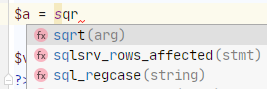Way cool! I don't have to remember what things are called, exactly. OK, I choose `sqrt()`, but I want more info. I hit Crl+Q: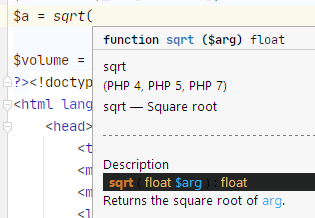Way way cool! PHPStorm shows me the complete docs for the function. Ahhhh! So good!

Let's go for way way way cool. What if I could get the same thing for `sphereVolume()`, the function I just wrote?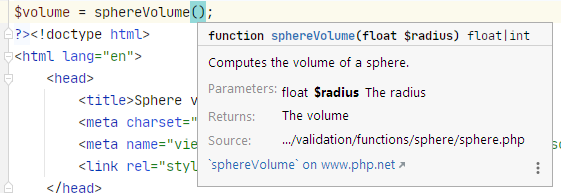Look at that! Tells me what the function does, what the params are, and the return value.

But that would be a crazy fever dream, right? How could the Stormster know about something I wrote?

Well, as you can tell from the screen shot, we can do that. Let's change the function to this:

1. /**
2.  * Computes the volume of a sphere.
4.  * @return float The volume
5.  */
8.     return \$volume;
9. }

The first five lines are comments. It's an alternate format to the usual `//`.

The comments for a "docblock." The first lines say what the function does. You can have an many lines in this description as you want.

For every parameter, we type in a line like this:

• * @param type \$name Description

`type` is the data type of the param. More in a mo. `\$name` is the param's name, and `Description` is what you think.

This...

• * @return type Description

... documents the return type.

This format for this docblock is called PHPDoc. There are similar formats for other languages.Ray

Why do this? If we wrote the function, we know what it does.

Good question. Every programmer makes their own libraries of functions. They often share their libraries with others. Documenting like this makes it easier for other people to use code you wrote. Big cost saving.

Job interview

This sounds good in a job interview: "I learned how to reduce costs by sharing code between developers."

We have coding standards in the course. One standard is that you must document each function. Check the coding standards page for all the deets.

Here's the code again:

1. /**
2.  * Computes the volume of a sphere.
4.  * @return float The volume
5.  */
8.     return \$volume;
9. }

These docs work best if they have data type information in them. `sphereVolume()` wants a number. Calling it like this...

• \$v = sphereVolume("Don't have a cow, man.");

.. won't work well.

I added `float` to lines 3, 4, and 6. `float` is the same as `Single` in VBA: a number that might have a decimal part.

The function definition of `sphereVolume()` says it takes a `float`:

What happens if you call it with a string? You can try it:

The 500 error means "something on the server is broken." So the `float` in...

... isn't a suggestion. It's mandatory. That's good! You don't want your program trying to do Weird Stuff with data.

1. /**
4.  * @return string Error message, MT if none.
5.  */
7.     \$errorMessage = '';
11.     }
12.     // Errors so far?
13.     if (\$errorMessage == '') {
14.         // Is radius big enough?
15.         if (\$radius <= 0) {
17.         }
18.     }
19.     // Return error message, if any.
20.     return \$errorMessage;
21. }

The data type in line 3 is `mixed`, meaning that we don't know what it is. Line 6 doesn't give a type for `\$radius`, either. The rest of the code should be easy for you to follow.

We'd call it like this:Wait, what if `radius` wasn't in the URL? Wouldn't there be an error?

Oooo, I'm impressed! Yes, we need an `isset()` check.

I could add it to `checkRadius()`, but I'm going to do it a little differently. The `isset()` check is something we should do for every GET param. I'm going to add a function to do the `isset()` check. I write the function once, but call it as much as I need. I save time.

Here's a call to the new function, followed by the function itself.

• // In the top...
•
• // Later...
•
• /**
•  * Get a value for parameter in the GET array.
•  * @param string \$paramName Name of the parameter.
•  */
• function getParamFromGet(string \$paramName) {
•     \$returnValue = null;
•     if (isset(\$_GET[\$paramName])) {
•         \$returnValue = \$_GET[\$paramName];
•         if (\$returnValue == '') {
•             \$returnValue = null;
•         }
•     }
•     return \$returnValue;
• }
•
• /**
•  * @return string Error message, MT if none.
•  */
• {
•     \$errorMessage = '';
•     // Missing?
•         \$errorMessage = "Sorry, radius is missing.<br>\n";
•     }
•     // Errors so far?
•     if (\$errorMessage == '') {
•         }
•     }
•     // Errors so far?
•     if (\$errorMessage == '') {
•         // Is radius big enough?
•         if (\$radius <= 0) {
•         }
•     }
•     // Return error message, if any.
•     return \$errorMessage;
• }

GET params are always strings. Even if you type a number at the end of a URL...

• page.php?length=66

... it will always be a string, as the debugger shows: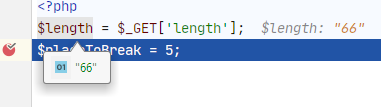The quotes tell you it's a string.

If you leave out the value, as in `page.php?length=`, you'll still get a string. MT: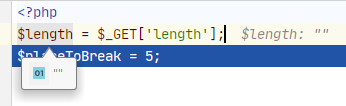Unless there isn't a `radius` in GETland, of course.

The rules can get a little confusing. The function `getParamFromGet()` always returns something predictable. Here it is again:

• /**
•  * Get a value for parameter in the GET array.
•  * @param string \$paramName Name of the parameter.
•  */
• function getParamFromGet(string \$paramName) {
•     \$returnValue = null;
•     if (isset(\$_GET[\$paramName])) {
•         \$returnValue = \$_GET[\$paramName];
•         if (\$returnValue == '') {
•             \$returnValue = null;
•         }
•     }
•     return \$returnValue;
• }

You get a string, if the parameter is there. You get null if the param is missing, or has no value (as in `page.php?length=`).

The function handles some messy details for you. Functions like this are useful, and turn up in many libraries.

### What's this null beast?

Here's some of the code:

• /**
•  ...
•  */
• function getParamFromGet(string \$paramName) {
•     \$returnValue = null;
•     ...
•     return \$returnValue;
• }

Null is a special value in PHP, and other languages. In most programs, it means "does not exist," although you can do with it what you want. In this course, let's stick with "does not exist."

Null is part of SQL, too. It's the standard way to show a field has no value. For example, a customer record might have a field called `birth_date`. To show that you don't know a particular customer's birth date, set the field to null.

Here's the function's code again:

• /**
•  * Get a value for parameter in the GET array.
•  * @param string \$paramName Name of the parameter.
•  */
• function getParamFromGet(string \$paramName) {
•     \$returnValue = null;
•     if (isset(\$_GET[\$paramName])) {
•         \$returnValue = \$_GET[\$paramName];
•         if (\$returnValue == '') {
•             \$returnValue = null;
•         }
•     }
•     return \$returnValue;
• }

If the param is in GETland, and has a value, you'll get a string from the function. If the param isn't there, you get null. That's what the comment shows:

The `|` means "or" in PHPDoc. So the function returns a string, or null.

### Back to price

Let's use what we learned, and make a price validation function. Here's the code from the last lesson.

• ...
• // Price.
• \$priceErrorMessage = '';
• if (!isset(\$_GET['price'])) {
•     \$priceErrorMessage = 'Sorry, you must give a price.<br>';
• }
• // Numeric check.
• if (\$priceErrorMessage == '') {
•     // Get the price.
•     \$price = \$_GET['price'];
•     // Check that price is numeric.
•     if (!is_numeric(\$price)) {
•         \$priceErrorMessage = 'Sorry, price must be a number.<br>';
•     }
• }
• // Range check.
• if (\$priceErrorMessage == '') {
•     if (\$price <= 0) {
•         \$priceErrorMessage = 'Sorry, price must be more than zero.<br>';
•     }
•     if (\$price >= 1000000) {
•         \$priceErrorMessage = 'Sorry, price is too high to be real.<br>';
•     }
• }
• ...

When I'm making a function, I often start by deciding how I want to call the function. That tells me what the function's params will be, and what I want to return.

Here's how I want to use the function `checkPrice()`:

• \$price = getParamFromGet('price');
• \$priceErrorMessage = checkPrice(\$price);

We already wrote `getParamFromGet()`, so just call it again.

`checkPrice()` will get a param that's a string, or null. How do I know? Because that's what `getParamFromGet()` returns:

• /**
•  * Get a value for parameter in the GET array.
•  * @param string \$paramName Name of the parameter.
•  */
• function getParamFromGet(string \$paramName) {
•     \$returnValue = null;
•     if (isset(\$_GET[\$paramName])) {
•         \$returnValue = \$_GET[\$paramName];
•         if (\$returnValue == '') {
•             \$returnValue = null;
•         }
•     }
•     return \$returnValue;
• }

So, with the code...

• \$price = getParamFromGet('price');
• \$priceErrorMessage = checkPrice(\$price);

... `checkPrice()` will get a string, or a null.

`checkPrice()` returns an error message. If it returns MT (''), there is no error.We could have it return null if there's no error, right?

Yes, but let's stick with MT, since we've been using that. Null would work just as well, though.

OK. We know the name of the function (`checkPrice()`), its params (string or null), and the return (a string, an error message or MT).

Let's wrap the code we have, clean it up, and add a `return`. Here's the whole thing:

• /**
•  * Check a price.
•  * @param mixed \$price The price to check.
•  * @return string Error message, MT if none.
•  */
• function checkPrice(\$price) {
•   \$priceErrorMessage = '';
•   // Check that price is numeric.
•   if (!is_numeric(\$price)) {
•       \$priceErrorMessage = 'Sorry, price must be a number.<br>';
•   }
•   // Range check.
•   if (\$priceErrorMessage == '') {
•       if (\$price <= 0) {
•           \$priceErrorMessage = 'Sorry, price must be more than zero.<br>';
•       }
•       if (\$price >= 1000000) {
•           \$priceErrorMessage = 'Sorry, price is too high to be real.<br>';
•       }
•   }
•   return \$priceErrorMessage;
• }Question. Price can't be zero, according to this code. What if want to give away some stuff? Where I work, we record some things with a price of zero.

Ooo, good point! The code we have wouldn't match your business policy. Let's change the code to handle that.

• /**
•  * Check a price.
•  * @param mixed \$price The price to check.
•  * @return string Error message, MT if none.
•  */
• function checkPrice(\$price) {
•   \$priceErrorMessage = '';
•   // Check that price is numeric.
•   if (!is_numeric(\$price)) {
•       \$priceErrorMessage = 'Sorry, price must be a number.<br>';
•   }
•   // Range check.
•   if (\$priceErrorMessage == '') {
•       if (\$price < 0) {
•           \$priceErrorMessage = 'Sorry, price must be zero or more.<br>';
•       }
•       if (\$price >= 1000000) {
•           \$priceErrorMessage = 'Sorry, price is too high to be real.<br>';
•       }
•   }
•   return \$priceErrorMessage;
• }Ethan

### Checking the state

Let's write a function to check the state, called like this:

• \$errorMessage = '';
• ...
• \$state = getParamFromGet('state');
• \$errorMessage .= checkState(\$state);

• /**
•  * Check the state.
•  * @param mixed \$state State from user.
•  * @return string Error message, MT if OK.
•  */
• function checkState(\$state) {
•
•     return \$errorMessage;
• }

The function has a name, a param, and a return value.

Now just pour in the filling.Yummy filling

• /**
•  * Check the state.
•  * @param mixed \$state State from user.
•  * @return string Error message, MT if OK.
•  */
• function checkState(\$state) {
•     \$errorMessage = '';
•     \$state = strtoupper(trim(\$state));
•     // Is the state known?
•     if (\$state != 'MI' && \$state != 'IL') {
•         \$errorMessage = "Sorry, '\$state' is not a recognized state.<br>";
•     }
•     // Return results.
•     return \$errorMessage;
• }

### Simple is good

Notice how much simpler the calling code is. The code at the start of the page is:

• \$price = getParamFromGet('price');
• \$priceErrorMessage = checkPrice(\$price);
• \$state = getParamFromGet('state');
• \$stateErrorMessage = checkState(\$state);
• \$errorMessage = \$priceErrorMessage . \$stateErrorMessage;
• ...
• ?><html ...

Moving validation into functions simplifies the code a lot. We can also reuse the validation functions on other pages.

BTW, we could also do...

• \$errorMessage = '';
• \$price = getParamFromGet('price');
• \$errorMessage .= checkPrice(\$price);
• \$state = getParamFromGet('state');
• \$errorMessage .= checkState(\$state);
• ...
• ?><html ...

Either way.Ethan

Wait, wouldn't error messages get erased?

Oh, never mind. I just noticed the . in:

• \$errorMessage .= checkPrice(\$price);

The . means append, so it's accumulating error messages.

That's right. Wouldn't work without the .

### Discount rate

OK, say the boss wants us to add a discount rate to the page. You can try it.The boss

http://webappexamples.skilling.us/validation/functions/multifield-with-functions/multifield-with-functions.php?state=mi&price=100&discount_rate=.1

Here's the validation function.

• /**
•  * Check a discount rate.
•  * @param mixed \$discountRate The discount rate to check.
•  * @return string Error message, MT if none.
•  */
• function checkDiscountRate(\$discountRate) {
•     \$errorMessage = '';
•     // Check that discount rate is numeric.
•     if (!is_numeric(\$discountRate)) {
•         \$errorMessage = 'Sorry, discount rate must be a number.<br>';
•     }
•     // Range check.
•     if (\$errorMessage == '') {
•         if (\$discountRate < 0) {
•             \$errorMessage = 'Sorry, discount rate must be zero or more.<br>';
•         }
•         if (\$discountRate >= 0.99) {
•             \$errorMessage = 'Sorry, discount rate is too high to be real.<br>';
•         }
•     }
•     return \$errorMessage;
• }

Here's the first part of the PHP file.

• <?php
• // Set up vars to track results.
• \$errorMessages = ''; // Composite error messsage.
• \$taxRate = 0; // Tax rate for a state.
• \$stateShortName = '';
• \$stateLongName = '';
• \$price = 0; // List price.
• \$discountRate = 0; // Discount rate, e.g., 0.1
• \$discountedPrice = 0; // Price after discount taken.
• \$tax = 0; // Tax, price * rate.
• \$total = 0; // Total after tax.
• // Check the state.
• \$stateShortName = getParamFromGet('state');
• \$stateErrorMessage = checkState(\$stateShortName);
• // Check the price.
• \$price = getParamFromGet('price');
• \$priceErrorMessage = checkPrice(\$price);
• // Check the discount rate.
• \$discountRate = getParamFromGet('discount_rate');
• \$discountRateErrorMessage = checkDiscountRate(\$discountRate);
• // Combine the error messages.
• \$errorMessages = \$stateErrorMessage . \$priceErrorMessage . \$discountRateErrorMessage;
• // Should there be processing?
• if (\$errorMessages == '') {
•     // No errors.
•     if (\$stateShortName == 'mi') {
•         \$taxRate = 0.06;
•         \$stateLongName = 'Michigan';
•     }
•     else {
•         \$taxRate = 0.0625;;
•         \$stateLongName = 'Illinois';
•     }
•     \$discount = \$price * \$discountRate;
•     \$discountedPrice = \$price - \$discount;
•     \$tax = \$discountedPrice * \$taxRate;
•     \$total = \$discountedPrice + \$tax;
• }
• ?><!doctype html>

The get-input-and-validate code is three lines per param, and one of those is a comment:

• // Check the THING.
• \$THING = getParamFromGet('THING PARAM');
• \$sTHINGErrorMessage = checkTHING(\$THING);

We'll be able to write new pages quickly, because we can reuse the functions.

Principle

Reuse your code across programs, using functions and page components.

### Variable scope

We want to reuse functions in different programs. We want to be able to drop a function we already wrote into a new program, and not have to worry that the function will mess up the new code, or the new code will mess up the function.

Variable scoping helps with that. Functions can't access variables outside themselves. They can only access data passed in through parameters.

(There's an exception to this. We'll see it later.)

Here's some code:

1. // Could be Area 51.
2. \$area = \$_GET['sales_region'];
3. \$length = \$_GET['length'];
4. \$vol = cubeVolume(\$length);
5. ...
6. <p>Region: <?= \$area ?></p>
7. <p>Cube volume: <?= \$vol ?></p>
8. ...
9.
10. function cubeVolume(\$length) {
11.     \$area = \$length * \$length;
12.     \$volume = \$area * \$length;
13.     return \$volume;
14. }

The function uses `\$area` inside it. You can't tell that without looking at the code, though. The main program uses `\$area` as well, for something else.

Remember, a variable points to a location in memory. If `\$area` in the main program, and `\$area` in the function, pointed to the same memory location, this program wouldn't work. The function would interfere with the main program.

What if there was a dozen functions? Reusing functions across programs would be a lot harder. You'd have to inspect the code for duplicate variable names, not only when you wrote the program for the first time, but every time anyone changed the program.

That would suck.

PHP builds a wall around each function.The only data that gets in, is the params. The only data that gets out, is the return value.

(Except for globals - more later.)

Each time PHP runs the function, it makes a function workspace. It creates `\$area` in the workspace. It's temporary. It points to a different location from the main program's `\$area`.

`\$area` is called a local variable. It's local to the function.

When the function exits, PHP destroys the workspace, including all the variables in it.

This applies to every function.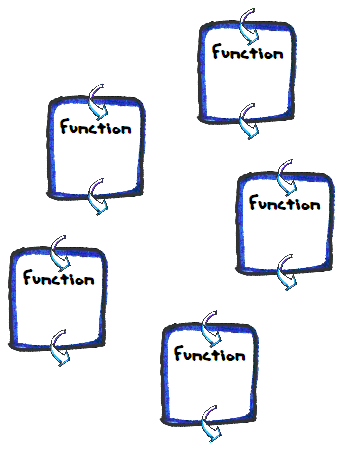Each has its own workspace. The workspaces are separate from the main program, and from each other. It doesn't matter what the variables in the functions are called. They won't interfere with anything.

In fact, it doesn't matter what the params are called, either. Check this out:

1. \$rectLength = \$_GET['rect_len'];
2. \$rectWidth = \$_GET['rect_wid'];
3. \$rectArea = rectangleArea(\$rectLength, \$rectWidth);
4. \$cubeLength = \$_GET['cube_len'];
5. \$cubeVolume = cubeVolume(\$cubeVolume);
6. ...
7. function cubeVolume(\$length) {
8.     \$area = \$length * \$length;
9.     \$volume = \$area * \$length;
10.     return \$volume;
11. }
12.
13. function rectangleArea(\$length, \$width) {
14.     \$area = \$length * \$width;
15.     return \$area;
16. }

This is just fine. `\$length` is used in both functions. They won't interfere with each other.

Think of the params names in a function as placeholders for values.

1. function cubeVolume(PLACE_HOLDER1) {
2.     \$area = PLACE_HOLDER1 * PLACE_HOLDER1;
3.     \$volume = \$area * PLACE_HOLDER1;
4.     return \$volume;
5. }
6.
7. function rectangleArea(PLACE_HOLDER1, PLACE_HOLDER2) {
8.     \$area = PLACE_HOLDER1 * PLACE_HOLDER2;
9.     return \$area;
10. }

The actual names of the params don't matter. They're stand-ins for values. As long as `cubeVolume` gets one value, it's happy. As long as `rectangleArea` gets two, it's happy.

The values don't have to be from variables, either. This is fine:

• \$rectArea = rectangleArea(\$prodDim*2, 17);

Two values, one from an expression, one a constant. Just fine.

Another:

• \$rectArea = rectangleArea(cubeVolume(\$erica)*2, cubeVolume(\$jane)*7);

I might break the expressions out, myself, but the code would work.

### Exercise

Exercise

Goat fuel

Cthulhu sponsors goat races every month. The course varies in length, and whether there are hurdles or not. Before each race, Cthulhu feeds the goats, so they have the energy they'll need. Write a program to work out the number of kilocalories needed for a race.

You can try my solution:

http://webappexamples.skilling.us/validation/exercises/goat-fuel/goat-fuel.php?goats=12&course_length=100&hurdles=n

The user types in some GET params:

• `goats`: The number of goats (must be a number more than zero)
• `course_length`: The course length (must be a number of at least 10)
• `hurdles`: Whether there are hurdles (must be y or n, allow upper- or lowercase)

Validate the data, showing all appropriate error messages. For example: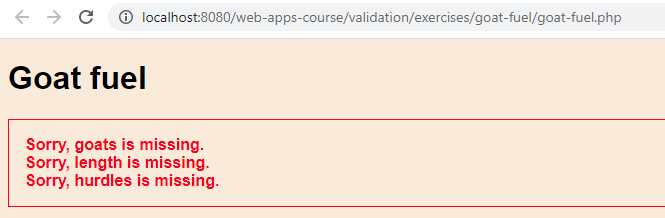Style as shown.

Sample output: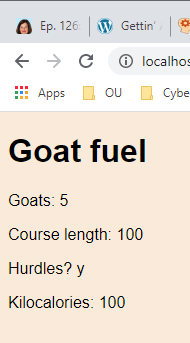Kilocalories is goats times length times 100 divided by 1,000. If there are hurdles, multiply kilocalories by two.

If more than 100 kilocalories are needed, Cthulhu wants you to bring in Euphonites, his goat nutritionist. Show a message to that effect: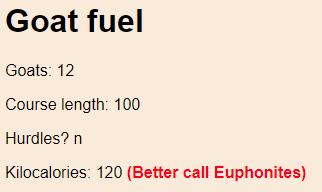You must use this code, with no changes:

• <?php
• // Init error message.
• \$errorMessage = '';
• // Validate goats.
• \$goats = getParamFromGet('goats');
• \$errorMessage .= checkGoats(\$goats);
• // Validate course length.
• \$courseLength = getParamFromGet('course_length');
• \$errorMessage .= checkCourseLength(\$courseLength);
• // Validate hurdles.
• \$hurdles = getParamFromGet('hurdles');
• \$errorMessage .= checkHurdles(\$hurdles);
• if (\$errorMessage == '') {
•     // All input valid. Compute kilocalories.
•     \$kiloCalories = \$goats * \$courseLength * 100 / 1000;
•     // Normalize \$hurdles.
•     \$hurdles = strtolower(trim(\$hurdles));
•     if (\$hurdles == 'y') {
•         \$kiloCalories *= 2;
•     }
• }
• ?><!doctype html>

Put your functions at the bottom of the page, or in a separate file.

Submit your URL, and a zip of all your files. The usual coding standards apply.

### Up next

Let's put our functions into a library file.